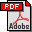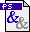Generic algorithm for 0-1 sorting Authors: Gianni Franceschini and Jyrki Katajainen Publication: CPH STL Report 2006-5, Department of Computer Science, University of Copenhagen (2006), 16 pp. Full text:PDF (193 kB)PS (403 kB) Abstract: In the 0/1-sorting problem, given a sequence S of elements drawn from a universe E and a characteristic function f: E → {0,1}, the task is to rearrange the elements in S so that every element x, for which f(x) = 0, is placed before any element y, for which f(y) = 1. Moreover, this reordering should be done stably without altering the relative order of elements having the same f-value, and space efficiently using only O(1) words of extra space. In this paper we present a generic algorithm for solving the 0/1-sorting problem which works optimally for many different kinds of sequences and characteristic functions. The model of computation used is a word RAM with a two-level memory hierarchy consisting of an ideal cache and an arbitrarily large main memory. The performance of our algorithm can be summarized as follows: Let n denote the length of S. The algorithm performs at most O(n) element exchanges, invocations of f, and word operations. Let w f (u) denote the amount of work done when applying f to element u. When the cost of the evaluation of the characteristic function is not uniform, but depends on the element f is applied to, the algorithm uses O(∑u∈ S w f (u)) work under the assumption that element exchanges involve O(1) work. Let B denote the size of cache blocks measured in elements. The algorithm incurs O(n / B) cache misses in the ideal-cache model under the tall-cache assumption (excluding the cache misses possibly generated by the invocations of f). Interestingly, our algorithm is neither aware of the characteristics of the cache (hardware obliviousness) nor the implementation of f (software obliviousness). BibLATEX: ```@report{FK2006R, author = {Gianni Franceschini and Jyrki Katajainen}, title = {Generic algorithm for 0-1 sorting}, type = {CPH STL Report}, number = {2006-5}, institution = {Department of Computer Science, University of Copenhagen}, year = {2006}, pagetotal = {16}, } ```